Analysis WebNotes
arrow_back arrow_forward

# Class Contents

## Recap

Remember right at the end of the last section, we had the axioms for the real numbers stated:
The real numbers are an ordered field with the least upper bound property.
There are two problems with this:

• First, we don't know that anything actually exists to meet these two conditions. The axiom list is a sort of "wish list" right now---it might be that we're asking for more than is possible.
• Equally disturbing, but in the other direction, it might be that there are many different things which satisfy these two axioms. How could we know we were all thinking of the "same" real numbers then?
These two problems are called problems of existence and uniqueness respectively. They are answered by the following theorem, which, although it needs to be proved, we shall take on faith for the moment.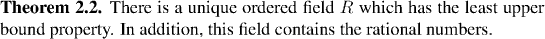Proving this theorem is certainly necessary for a proper development of analysis. On the other hand it's also hard work, and so we'll delay it until after we've seen more of how the properties it identifies for the real numbers are used.

Back in the Introduction we tried to prove the Archimedean Property of the real numbers. Back then, it was an illustration of how we needed firm principles of what we could and could not do with real numbers. Now that we have them, our first business should be to prove this theorem: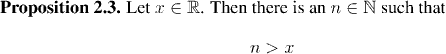This result has an important corollary: that between any two real numbers there always lies a rational number. In other words (we shall make this precise later on) the rational numbers are dense in the reals.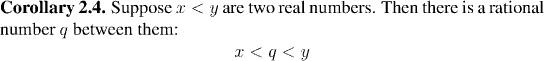We see from this corollary that the rationals are interwoven with the reals. Yet we haven't seen yet that there are any more real numbers than there are rationals. Back in the last section we asserted that the rational numbers do not have the least upper bound property, and the reals do have this property. It is now time to show that the rationals do not have the least upper bound property, and also that there are real numbers which are not rational.

## Irrational Numbers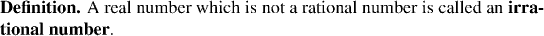It's all very well to make this definition, but we don't yet know that there are any irrational numbers. In fact there are (as we'll see later on in the course) far more irrational numbers than rationals. However, for the moment, we'll just give an example of one irrational number; the square root of 2.

We start off by proving a very old fact (attributed to a student of Pythagoras) that there is no rational number which squares to 2. Of course, at that point we don't yet know for sure that there is a real number which squares to 2, but we prove that next. The fact that the rational numbers do not have the least upper bound property will also drop out of our work here.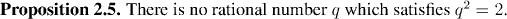Now we can prove that there is a real number whose square is 2 (so that "the square root of 2" really does exist). The way we get this is by showing that the supremum of the set of positive rational numbers which square to less than 2 does itself square to exactly 2. In view of Proposition 2.5, that set can't possibly have a supremum in the rationals. (In fact there's a tiny bit more to it than that. See below.)

Example: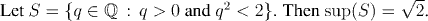## The Greatest Lower Bound of a Set

We've thought a lot about upper bounds and the least upper bound of a set. What about lower bounds and the greatest lower bound (which is the corresponding notion to the least upper bound).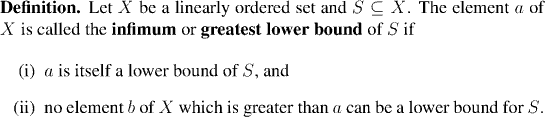One of the axioms of the real numbers was that

whenever a non-empty set of real numbers is bounded above, it has a supremum.
The corresponding fact for infimums does not need its own axiom: it can be proved using the least upper bound property.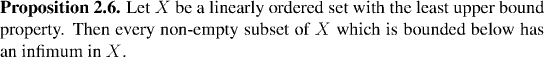Factoid:

The rational numbers do not have the least upper bound property.

Strictly speaking, in order to say that the rational numbers don't have the least upper bound property, we should take the set of positive rationals which square to less than 2, observe that it is non-empty and bounded above, and then suppose that it has a rational supremum, say a. The claim will then follow when we get a contradiction.

Since a is an upper bound for the set in the rationals, it is also an upper bound for the set in the reals, and so the square root of 2 is less than or equal to it. However a cannot be equal to the square root of 2, by Proposition 2.5, so a is greater than root 2. By Corollary 2.4 there is another rational number between root 2 and a. This number must also be an upper bound for our set (since it is greater than root 2), which contradicts a being the least upper bound.